# 群晖nas自建企业微信并配置异常推送通知

## 效果图展示## 创建企业微信

http://work.weixin.qq.com/CorpID和Secret、Agentld


## 创建PHP Send发送脚本

<?php
//i4t.com 群晖nas发送告警

$num =$_GET['num'];
$passwd =$_GET['passwd'];
$title =$_GET['title'];
$description =$_GET['description'];

// 获取 access_token
function getToken(){

// 定义 id 和 secret
$corpid='wwc13*******';//这里填写你的企业 ID$corpsecret='f2MBU****asdx***-asd**';//这里填写你刚创建的应用 secret

// 读取 access_token
include './access_token.php';

// 判断是否过期
if (time() > $access_token['expires']){ // 如果已经过期就得重新获取并缓存$access_token = array();
$access_token['access_token'] = getNewToken($corpid,$corpsecret);$access_token['expires']=time()+7000;

// 将数组写入 php 文件
$arr = '<?php'.PHP_EOL.'$access_token = '.var_export($access_token,true).';'.PHP_EOL.'?>';$arrfile = fopen("./access_token.php","w");
fwrite($arrfile,$arr);
fclose($arrfile); // 返回当前的 access_token return$access_token['access_token'];

}else{

// 如果没有过期就直接读取缓存文件
return $access_token['access_token']; } } // 获取新的 access_token function getNewToken($corpid,$corpsecret){$url = "https://qyapi.weixin.qq.com/cgi-bin/gettoken?corpid={$corpid}&corpsecret={$corpsecret}";
$access_token_Arr = https_request($url);
return $access_token_Arr['access_token']; } // curl 请求函数 function https_request ($url){
$ch = curl_init(); curl_setopt($ch, CURLOPT_URL, $url); curl_setopt($ch,CURLOPT_RETURNTRANSFER,1);
curl_setopt($ch, CURLOPT_SSL_VERIFYPEER, false);$out = curl_exec($ch); curl_close($ch);
return  json_decode($out,true); } // 发送应用消息函数 function send($data){
$ch = curl_init(); curl_setopt($ch, CURLOPT_URL, 'https://qyapi.weixin.qq.com/cgi-bin/message/send?access_token='.getToken());
curl_setopt($ch, CURLOPT_POST, true); curl_setopt($ch, CURLOPT_POSTFIELDS, $data); curl_setopt($ch, CURLOPT_SSL_VERIFYPEER, 0);
curl_setopt($ch, CURLOPT_SSL_VERIFYHOST, 2); curl_setopt($ch, CURLOPT_RETURNTRANSFER, true);
return curl_exec($ch); } // 文本卡片消息体$postdata = array(
'touser' => '@all',          //不需要写联系人，会发送给所有人
'msgtype' => 'textcard',
'agentid' => '1000004',//这里填写你刚创建的应用 AgentId
'textcard' => array(
'title' => $title, 'description' =>$description,
'num' => $num, 'url' => 'https://i4t.com', //这里的域名写不写都可以，可以写成群晖nas的域名 'passwd' =>$passwd,
),
'enable_id_trans' => 0,
'enable_duplicate_check' => 0,
'duplicate_check_interval' => 1800
);

// 调用发送函数
echo send(json_encode($postdata)); // echo "$title, $description" ?> 第二种: 文本推送 <?php$num = $_GET['num'];$passwd = $_GET['passwd'];$content = $_GET['title'];$msg = $_GET['description'];$url = $_GET['down.frps.cn']; function curlPost($url,$data=""){$ch = curl_init();
$opt = array( CURLOPT_URL =>$url,
CURLOPT_POST    => 1,
CURLOPT_POSTFIELDS      => $data, CURLOPT_RETURNTRANSFER => 1, CURLOPT_TIMEOUT => 20 );$ssl = substr($url,0,8) == "https://" ? TRUE : FALSE; if ($ssl){
$opt[CURLOPT_SSL_VERIFYHOST] = 1;$opt[CURLOPT_SSL_VERIFYPEER] = FALSE;
}
curl_setopt_array($ch,$opt);
$data = curl_exec($ch);
curl_close($ch); return$data;
}

$corpid="wwc13*****7c750d"; //企业id$corpsecret="f2MBU****3NPTHo_****-****_oFTvLk";       //企业secret
$Url="https://qyapi.weixin.qq.com/cgi-bin/gettoken?corpid=$corpid&corpsecret=$corpsecret";$res = curlPost($Url);$ACCESS_TOKEN=json_decode($res)->access_token;$Url="https://qyapi.weixin.qq.com/cgi-bin/message/send?access_token=$ACCESS_TOKEN";$data="{\"touser\":\"@all\",\"msgtype\":\"text\",\"agentid\":1000004,\"text\":{\"content\":\"$content\n$msg$url\"},\"safe\":0}"; //此处ID需要修改，1000004修改为自己应用id$res = curlPost($Url,$data);
$errmsg=json_decode($res)->errmsg;
if($errmsg==="ok"){ echo "发送成功！"; }else{ echo "发送失败，".$errmsg;
}
?>

## 配置Send脚本并测试http://send.frps.cn/index.php?num=777&passwd=123&title=i4t.com标题&description=内容

• [ ] num任意id (传参需要为群晖需要有电话号，实际上不显示)
• [ ] passwd任意密码 (传参需要)
• [x] title发送通知标题 (这里随便写，后面我们修改群晖的即可)
• [x] description 发送内容 (这里随便写，后面由群晖自己生成)## 配置群晖Nas通知• [x] 用户账号为title发送信息的标题，自己定义即可
• [ ] 密码随便写，什么密码都不是，只是需要这个值 (不可以不填)
• [ ] 手机号随便写，不可以不填## 配置应用通知## 测试！2022-5-4 3:18:15

## 群晖SSD高速缓存设置教程 添加SSD固态硬盘作为缓存盘

2022-5-9 12:06:19

26 条回复 A文章作者 M管理员
1. 短信测试发出但一直收不到

• 1.查看nginx日志，群晖如果发送短信会进行get请求
2.检查群晖消息推送配置是否都正确
3.群晖消息推送是否勾选消息已经推送，这2个都要勾选
4.下载个镜像测试，只要ui界面显示推送，那么群晖就可以收到。

截图部分需要勾选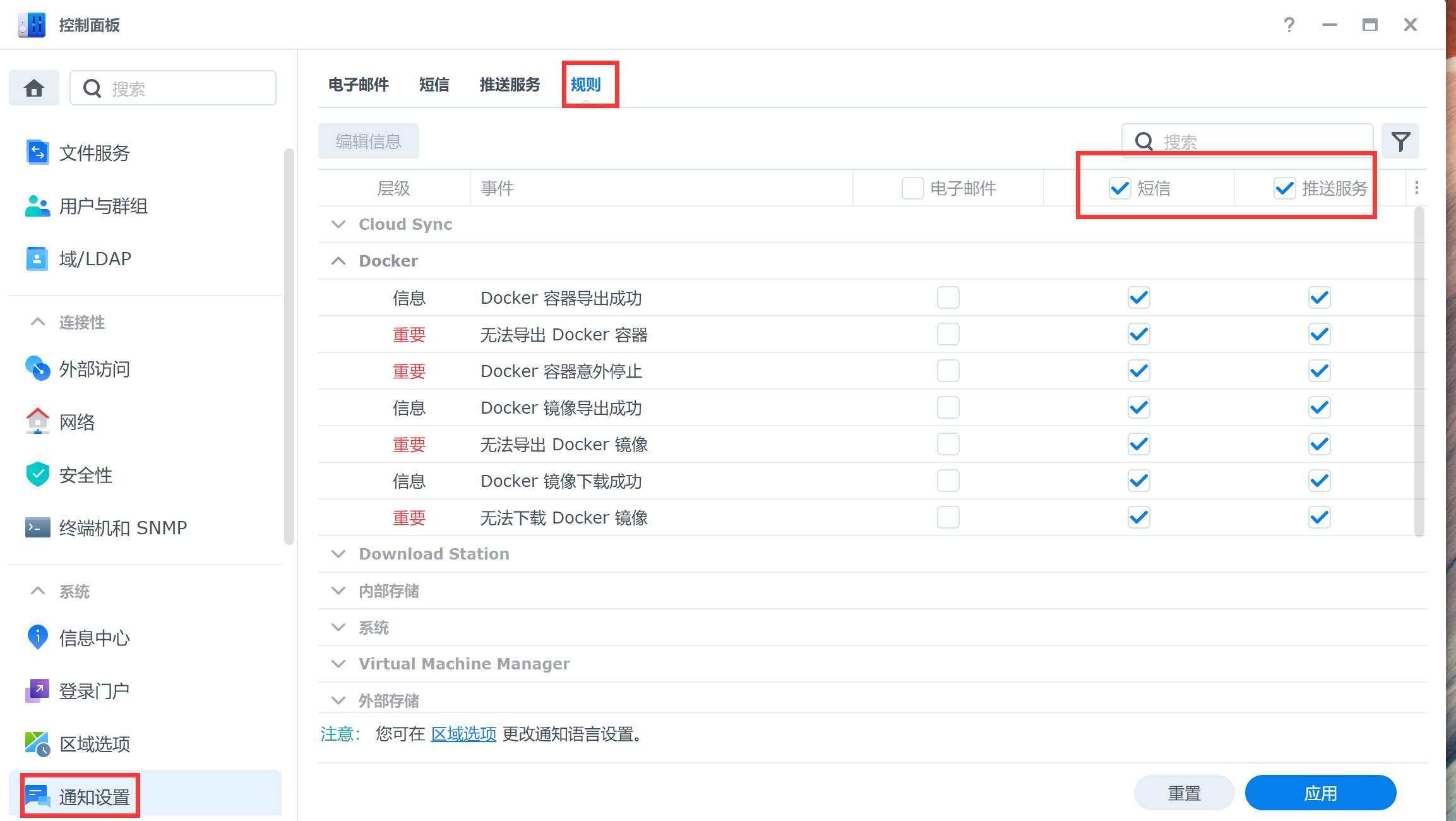• 直接测试就会提示命令运行失败[img]https://postimg.cc/cr9p09KR[/img]，直接应用在测试就提示已发送但收不到推送[img]https://postimg.cc/yJbxRXrc[/img]，这应该是群晖短息通知的问题吧

• 我还试了下bark测试也是同样的结果..

• 检查手动文件吧，我感觉是php这发送失败

• 我就是手动php全部成功才怀疑群晖问题的😥

• 运行失败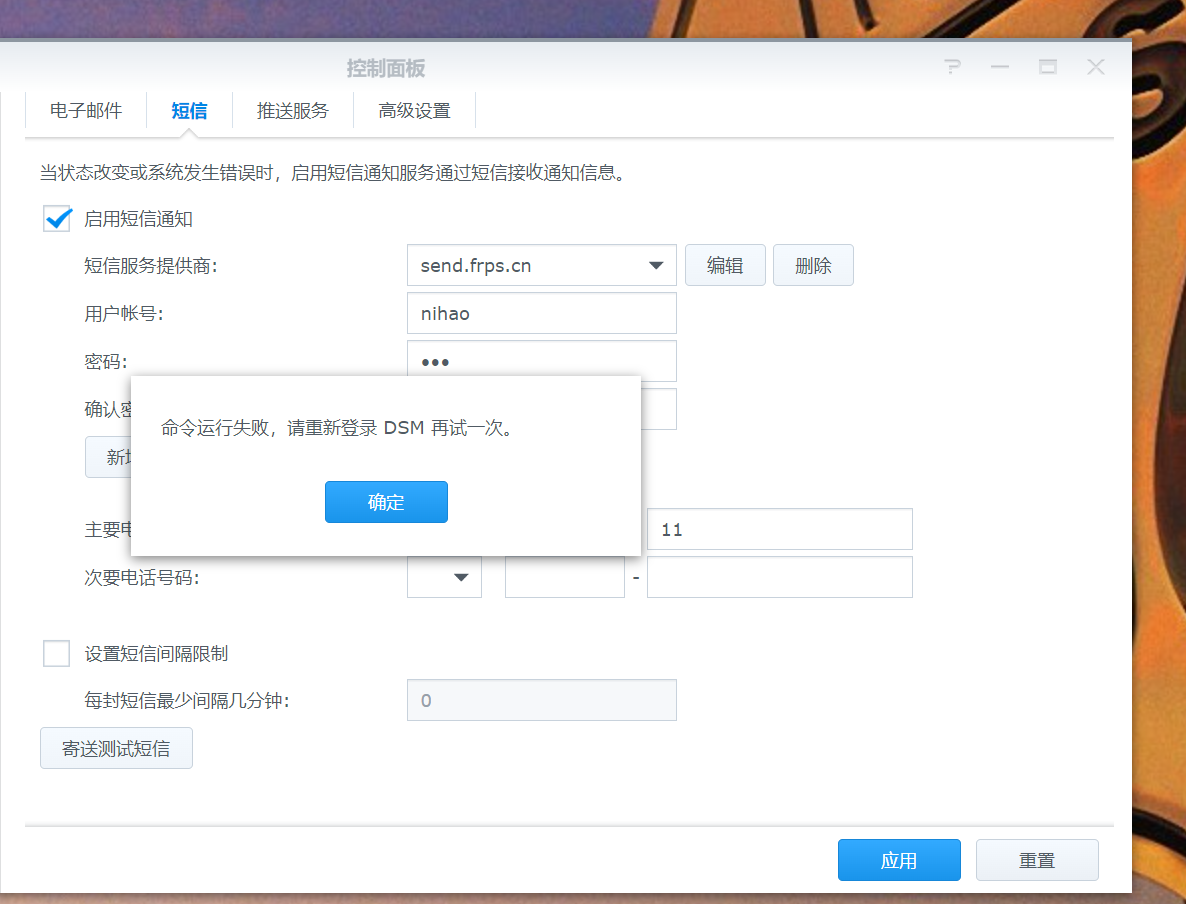• 这设置的没问题吧？😂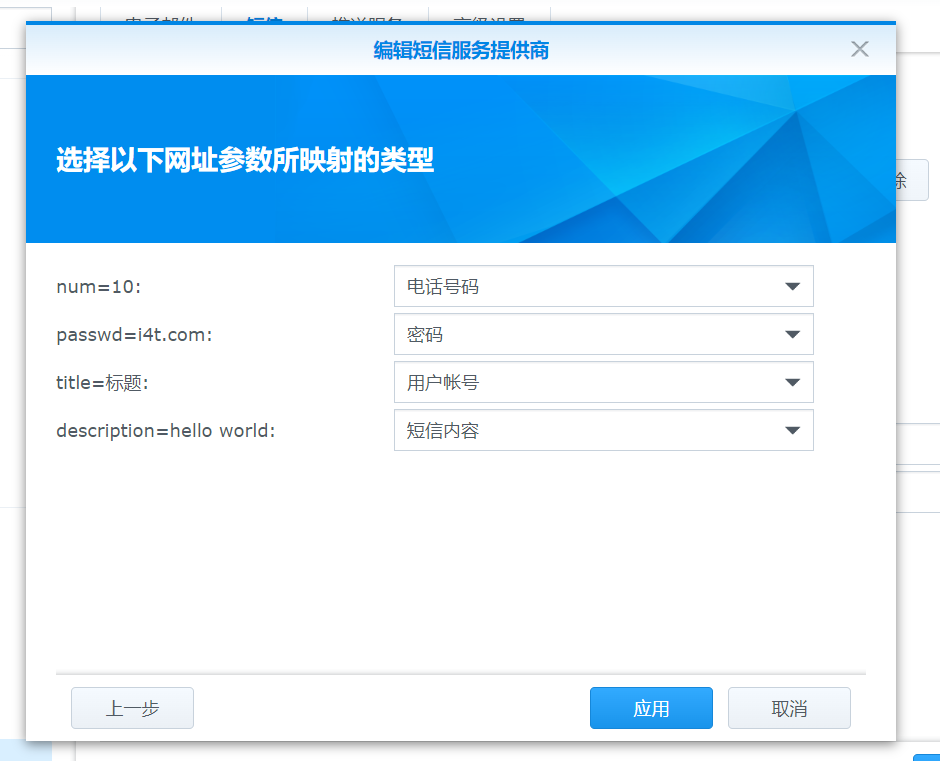• 我这里可以收到😁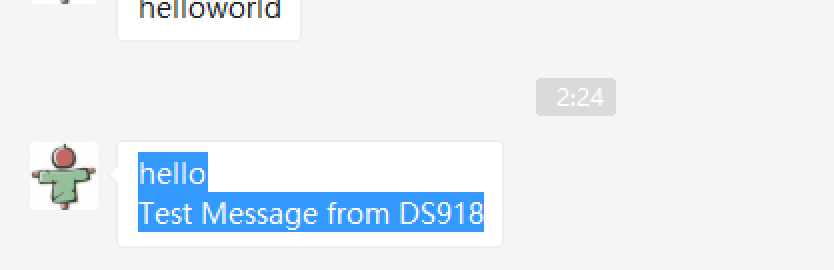• 😥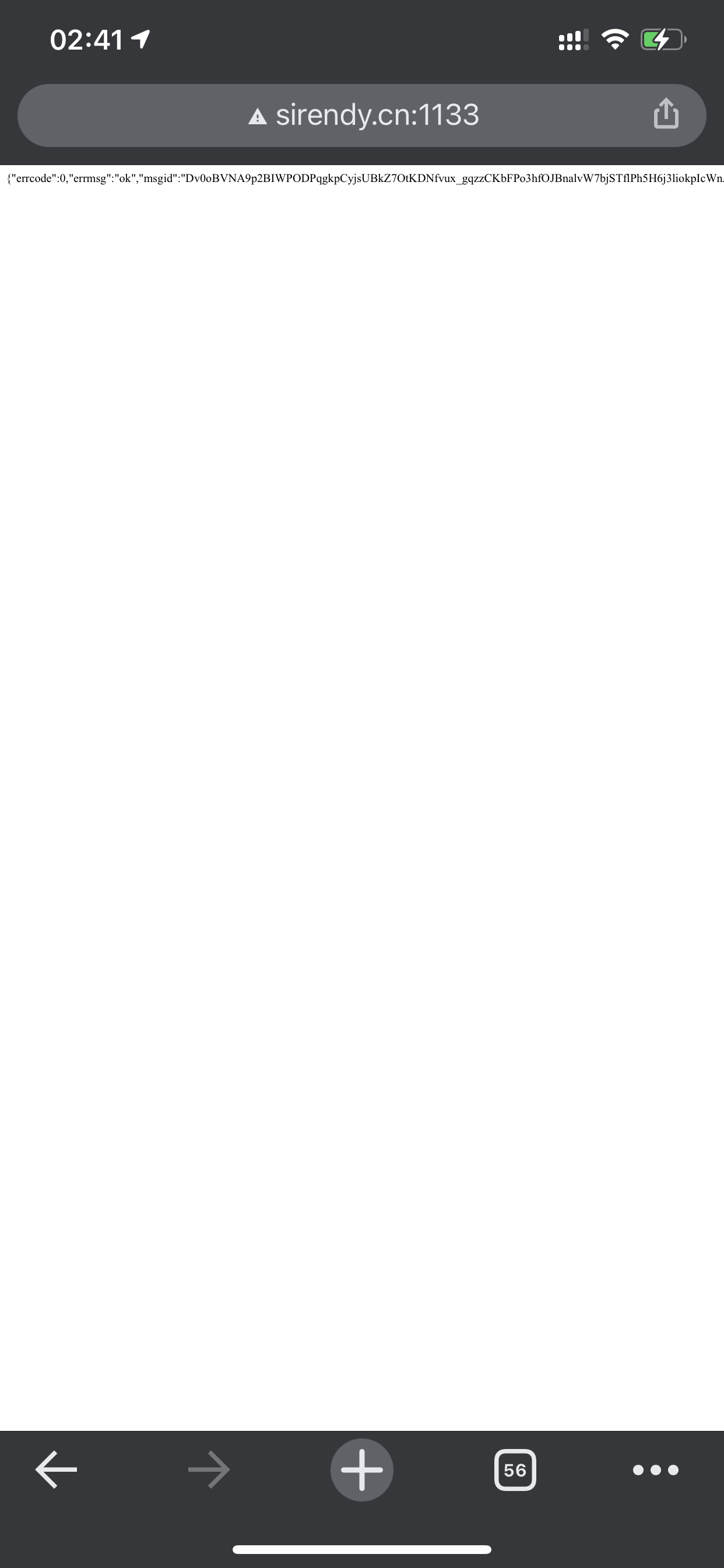• 你收到短信了吗？

• 我手动可以收到啊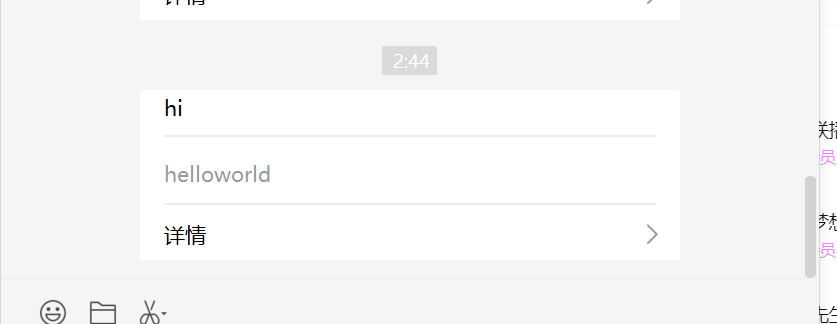• 就是群晖里设置就收不到，就一直运行失败

• 直接应用再测试提示已发送，但就是收不到推送！😂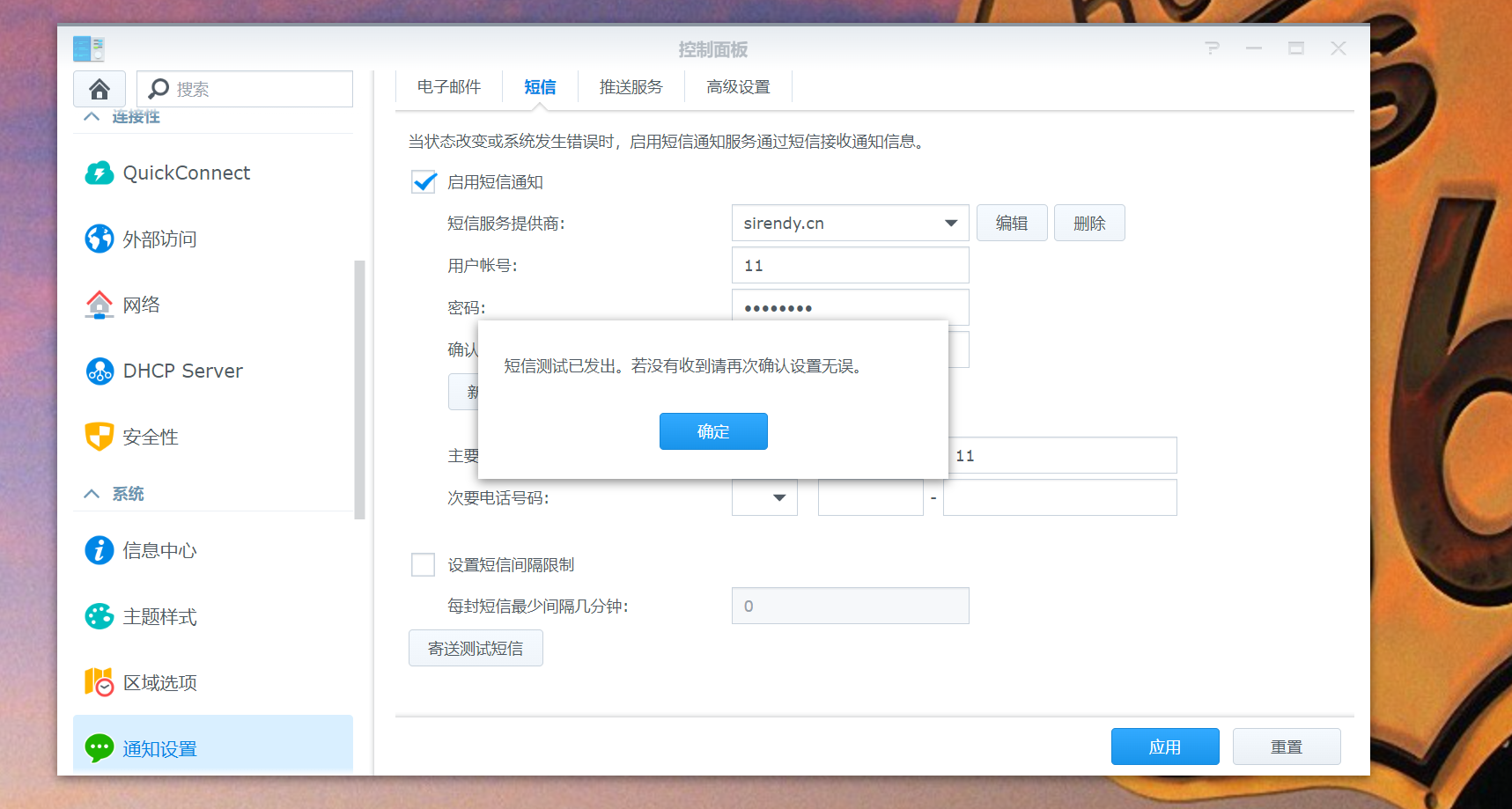• 把这个文件删除，然后在调用。顺便看一下nginx日志，会有请求信息

• 你检查一下这里的应用id对不对，如果这里设置错误，也是可以发送成功的。但是不是发给你了，@all啥的，你可以把配置文件贴出来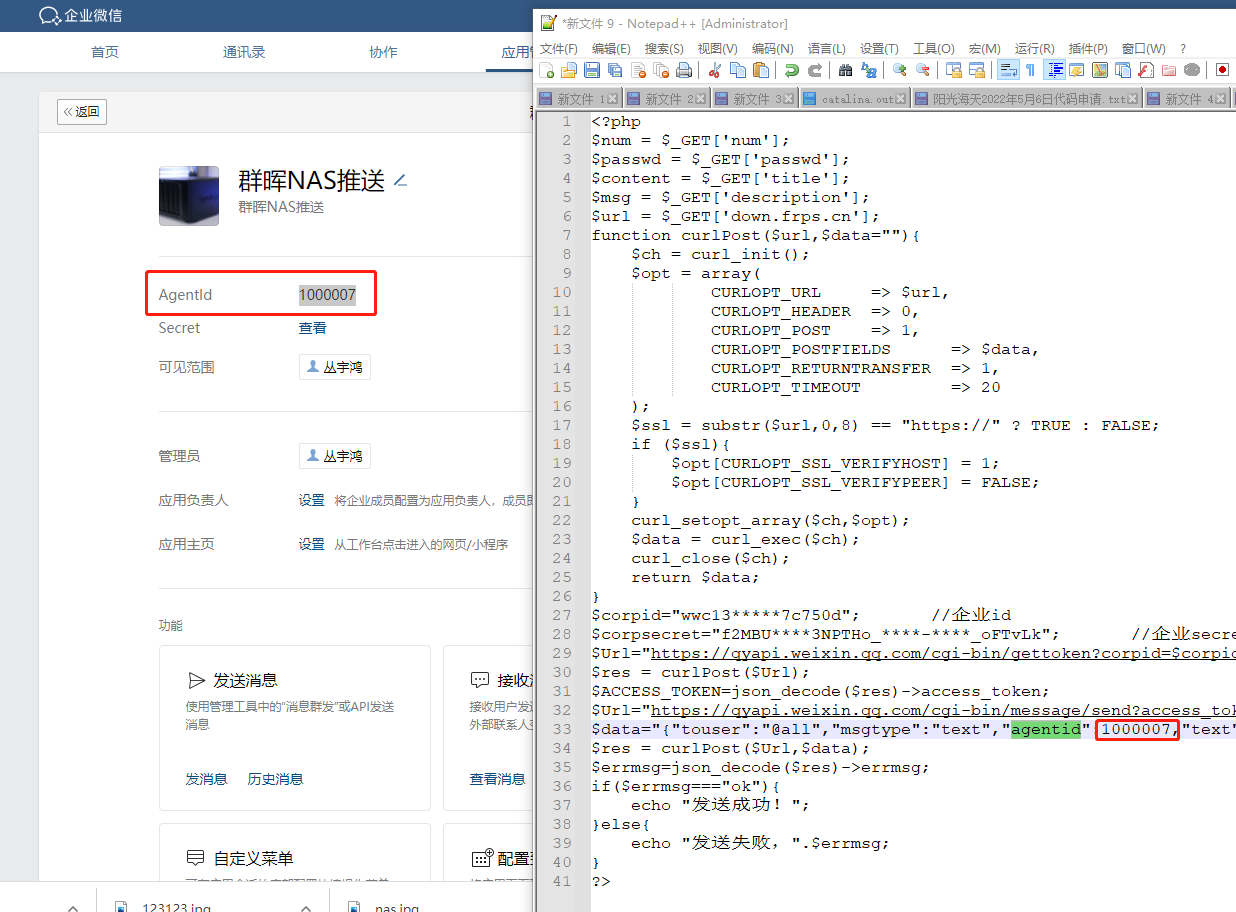• 手动能收到没道理会设置错误啊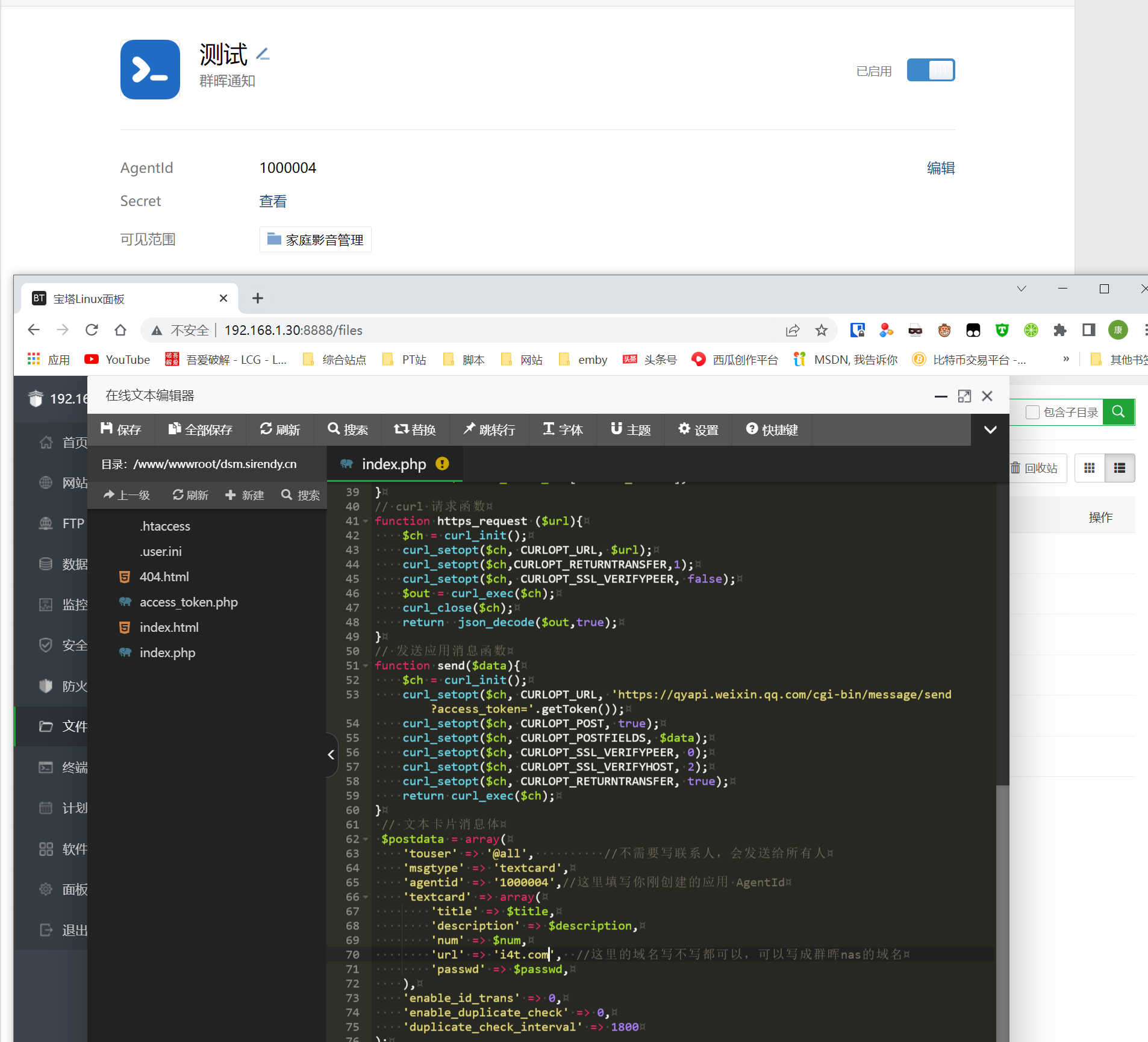• 这样，你把群晖配置界面都截图上传，我给你看一下。nginx日志在宝塔上也截图看一下

• 😥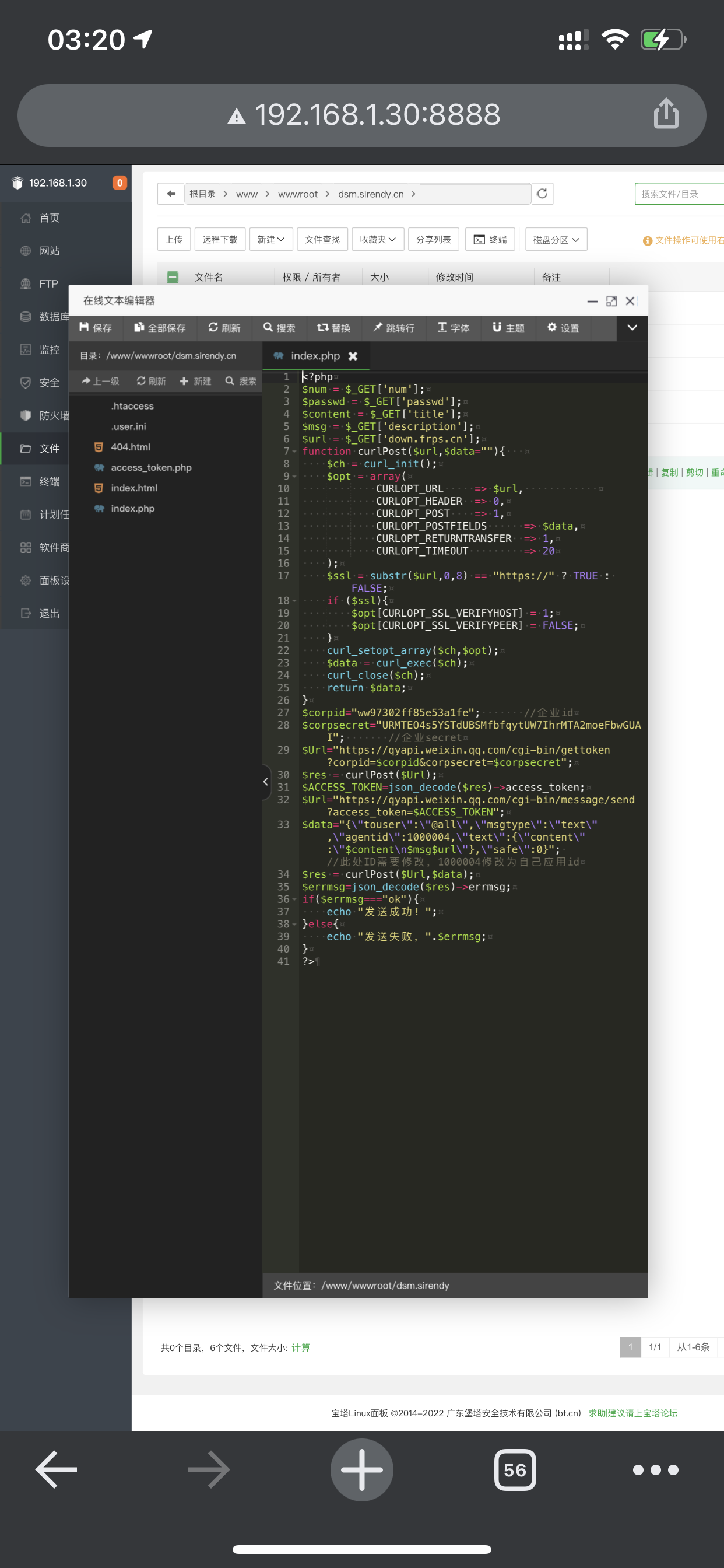•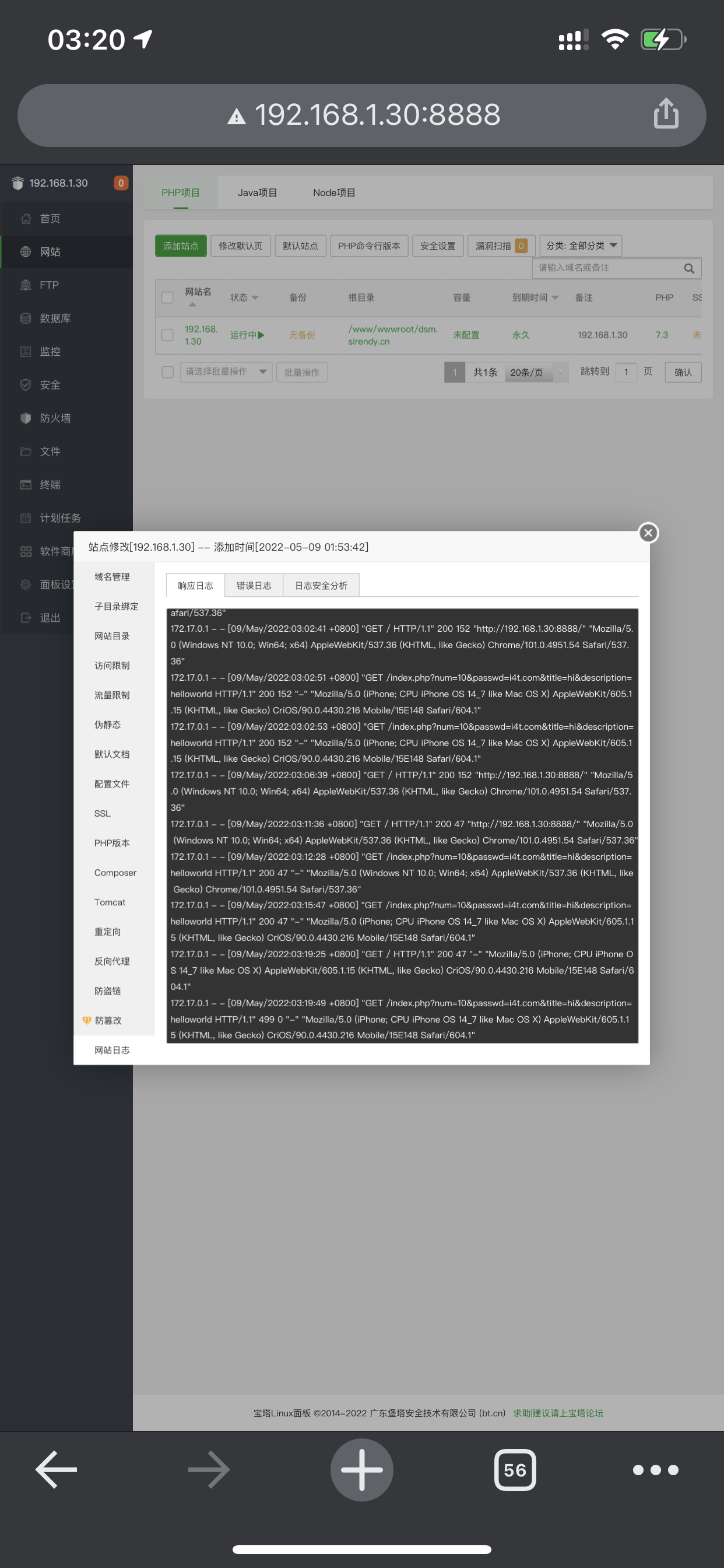• 😥• 手动两次都成功收到短信• 日志没反应• 😥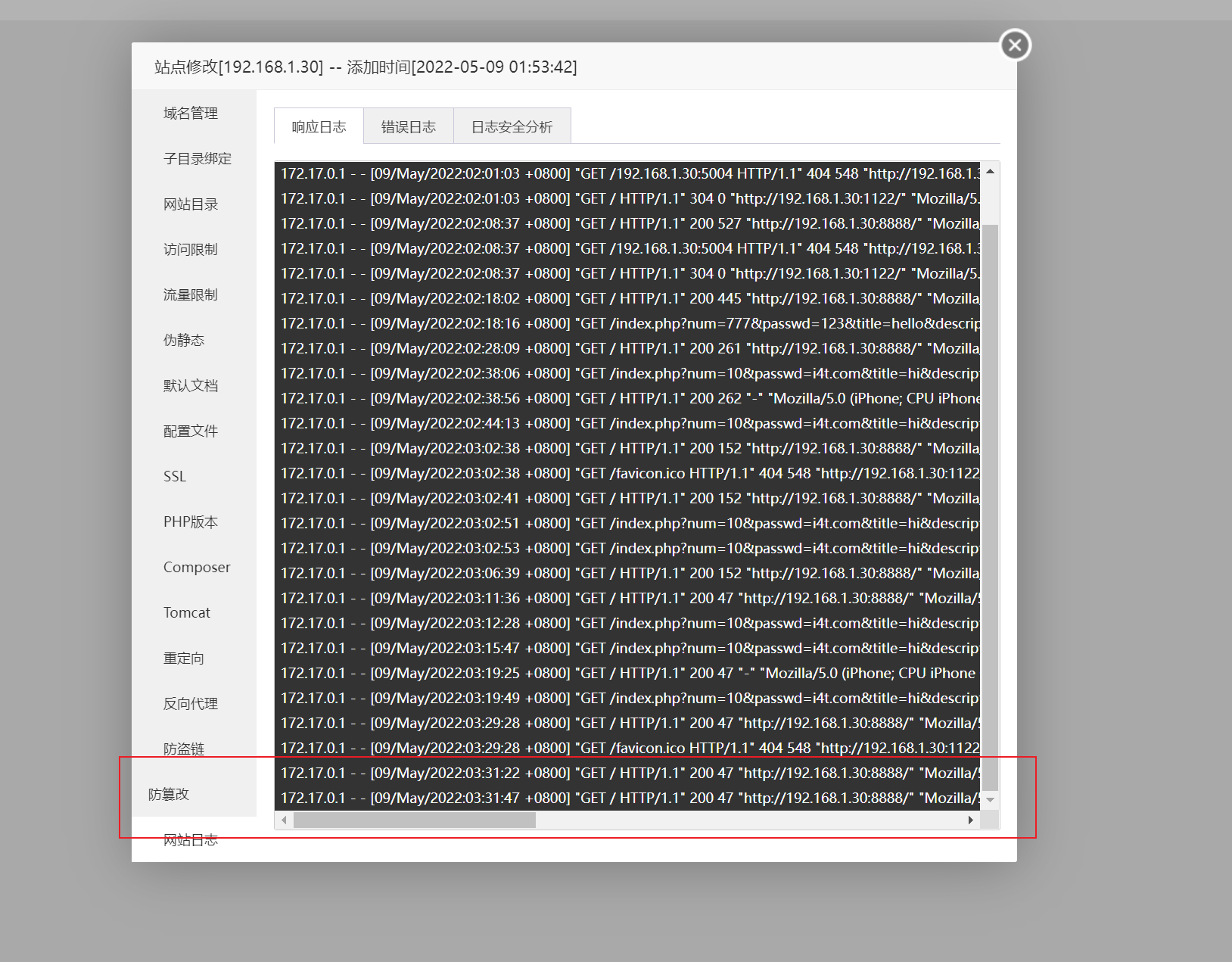• 上图所示，和群晖没啥关系了，你手动php发送试一下。
http://send.frps.cn/v1.php?num=10&passwd=i4t.com&title=标题&description=helloworld

http://你的域名或者ip/文件.php?num=10&passwd=i4t.com&title=标题&description=helloworld